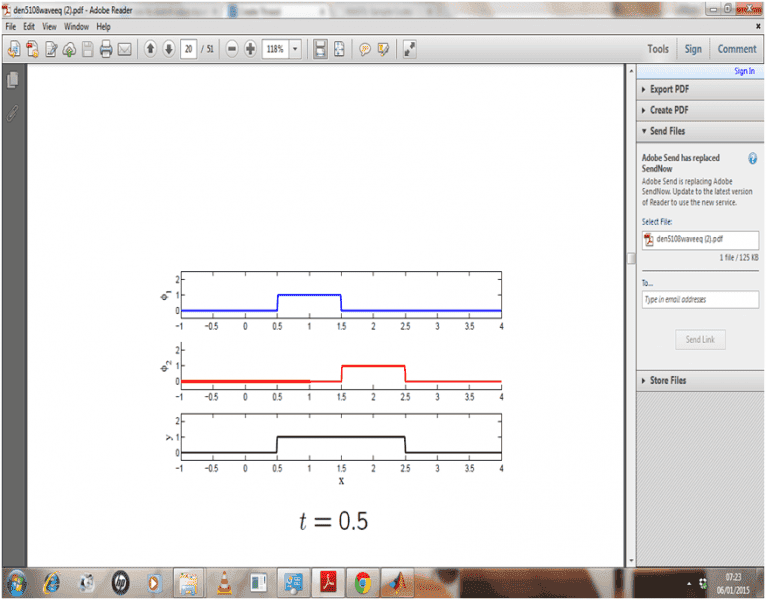# Help please in matlab -- plotting a D'Alembert wave equation

• MATLAB
Can someone please help me in plotting a D'Alembert wave equation solution in MATLAB? I am so confused as how to plot it in MATLAB

I need to plot a graph like the one belowjedishrfu
Mentor
It talks about plotting multiple lines.

If you're looking for more info like generating the data for plot then you'll need to find another tutorial.

It talks about plotting multiple lines.

If you're looking for more info like generating the data for plot then you'll need to find another tutorial.

No man I already know how to plot multiple lines....its on how to generate the data actually

Mark44
Mentor
No man I already know how to plot multiple lines....its on how to generate the data actually
Show us what you have already tried. Be advised, however, that we're not going to do your work for you...

Show us what you have already tried. Be advised, however, that we're not going to do your work for you...

What do you want to see? I have typed all sorts of commands in MATLAB getting nothing as a result so I have nothing to show you.

I tried x = [-1 -0.5 0 0.5...4] then did y = [0,0,0 placed 2 for all the x values in the picture that had y as 2] then did plot(x,y) but got some stupid graph

anyways I don't know how to use PDE commands in MATLAB unless someone wants to be generous and teach me...I am good at coding and maths I swear! I will learn fast.

Mark44
Mentor
What do you want to see?
Show us what you have tried.
JI567 said:
I have typed all sorts of commands in MATLAB getting nothing as a result so I have nothing to show you.

I tried x = [-1 -0.5 0 0.5...4] then did y = [0,0,0 placed 2 for all the x values in the picture that had y as 2] then did plot(x,y) but got some stupid graph

anyways I don't know how to use PDE commands in MATLAB unless someone wants to be generous and teach me...I am good at coding and maths I swear! I will learn fast.
PDE? Partial differential equations?

DrClaude
Mentor
I tried x = [-1 -0.5 0 0.5...4] then did y = [0,0,0 placed 2 for all the x values in the picture that had y as 2] then did plot(x,y) but got some stupid graph
To get straigth veritcal lines (by hand), you need to double the points at which the lines appear. For instance, you need two points at x = 0.5, one with y=0 followed by one with y=2.